1. /
2. CBSE
3. /
4. Class 08
5. /
6. Mathematics
7. /
8. NCERT Solutions for Class...

# NCERT Solutions for Class 8 Maths Exercise 4.3### myCBSEguide App

Download the app to get CBSE Sample Papers 2023-24, NCERT Solutions (Revised), Most Important Questions, Previous Year Question Bank, Mock Tests, and Detailed Notes.

NCERT solutions for Class 8 Maths Practical Geometry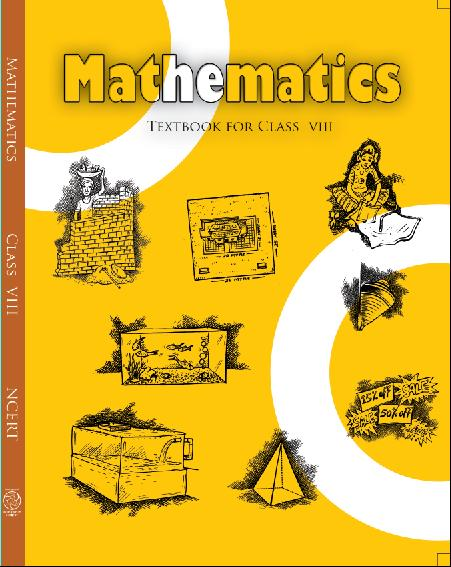## NCERT Solutions for Class 8 Maths Practical Geometry

###### 1. Construct the following quadrilaterals:

MO = 6 cm, OR = 4.5 cm,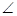M =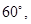O =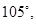R =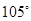PL = 4 cm, LA = 6.5 cm,P =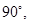A =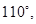N =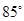(iii) Parallelogram HEAR

HE = 5 cm, EA = 6 cm,R =(iv) Rectangle OKAY

OK = 7 cm, KA = 5 cm

Ans.  (i) Given:  MO = 6 cm, OR = 4.5 cm,M =O =R =###### Steps of construction: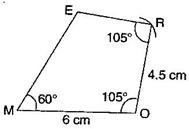(a) Draw a line segment MO = 6 cm.

(b) ConstructR =and taking radius 4.5 cm, draw an arc taking O as centre, which intersects at R.

(c) Also construct an angleat R and produce the side RE.

(d) Construct another angle of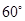at point M and produce the side ME. Both sides ME and RE intersect at E.

It is the required quadrilateral MORE.

(ii) Given:  PL = 4 cm, LA = 6.5 cm,P =A =N =###### To construct: A quadrilateral PLAN.

To findL =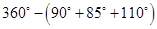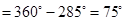Steps of construction: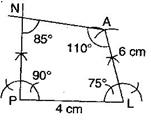(a) Draw a line segment PL = 4 cm.

(b) Construct angle of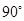at P and produce the side PN.

(c) Construct angle of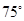at L and with L as centre, draw an arc of radius 6 cm, which intersects at A.

(d) ConstructA =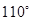at A and produce the side AN which intersects PN at N.

It is the required quadrilateral PLAN.

NCERT Solutions for Class 8 Maths Exercise 4.3

###### (iii)Given:  HE = 5 cm, EA = 6 cm,R =To construct: A parallelogram HEAR.

To findH =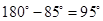[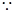Sum of adjacent angle of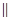gm is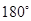]

Steps of construction: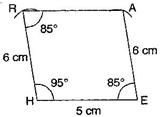(a) Draw a line segment HE = 5 cm.

(b) ConstructH =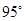and draw an arc of radius 6 cm with centre H. It intersects AR at R.

(c) Join RH.

(d) DrawR =E =and draw an arc of radius 6 cm with E as a centre which intersects RA at A.

(e) Join RA

It is the required parallelogram HEAR.

(iv) Given:  OK = 7 cm, KA = 5 cm

To construct: A rectangle OKAY.

NCERT Solutions for Class 8 Maths Exercise 4.3

###### Steps of construction: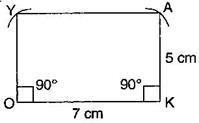(a)  Draw a line segment OK = 7 cm.

(b) Construct angleat both points O and K and produce these sides.

(c) Draw two arcs of radius 5 cm from points O and K respectively. These arcs intersect at Y and A.

(d) Join YA.

It is the required rectangle OKAY.

## NCERT Solutions for Class 8 Maths Exercise 4.3

NCERT Solutions Class 8 Mathematics PDF (Download) Free from myCBSEguide app and myCBSEguide website. Ncert solution class 8 Mathematics includes text book solutions from Class 8 Maths Book . NCERT Solutions for CBSE Class 8 Maths have total 16 chapters. 8 Maths NCERT Solutions in PDF for free Download on our website. Ncert class 8 solutions PDF and Maths ncert class 8 PDF solutions with latest modifications and as per the latest CBSE syllabus are only available in myCBSEguide.

## CBSE app for Class 8

To download NCERT Solutions for class 8 Social Science, Computer Science, Home Science,Hindi ,English, Maths Science do check myCBSEguide app or website. myCBSEguide provides sample papers with solution, test papers for chapter-wise practice, NCERT solutions, NCERT Exemplar solutions, quick revision notes for ready reference, CBSE guess papers and CBSE important question papers. Sample Paper all are made available through the best app for CBSE students and myCBSEguide website.Test Generator

Create question paper PDF and online tests with your own name & logo in minutes.myCBSEguide

Question Bank, Mock Tests, Exam Papers, NCERT Solutions, Sample Papers, Notes

### 7 thoughts on “NCERT Solutions for Class 8 Maths Exercise 4.3”

1. nyx…

2. in question no ii the length is 6.5 not 6

3. Hmm… seems ok to me……

4. It’s is very cool

5. Nice it’s very easy

6. Nice it’s very easy